### Electric Field Targets

 Electric Field Target allows to Minimize or to Maximize a squared modulus of the electric field amplitude $$|E|^2$$. Electric filed editor allows to specify Incident Angle Polarization Wavelength range Grid type (Even, Logarithmic, Inversely Proportional) Number of wavelength points Number of discretization points for every layer that should be considered. Example: It was demonstrated that by suppressing the electric field in high-index layers and shifting its maxima to low-index layers the damage threshold can be increased by 30%. The design of the coating with optimized electric field intensity (EFI). The laser central wavelength was 1030nm, the high reflectance range is from 960 to 1100 nm. Thin film materials are Ta2O5 and SiO2, substrate is Fused Silica.The target was a combination of two targets: (1) conventional one with target reflectance of 100% in the range from 960 to 1100 nm and (2) electric-field intensity target with minimized intensity in Ta2O5 layers (see above). Refractive index profile of a 35-layer Quarter-Wave Mirror, thin film materials are Ta2O5/SiO2: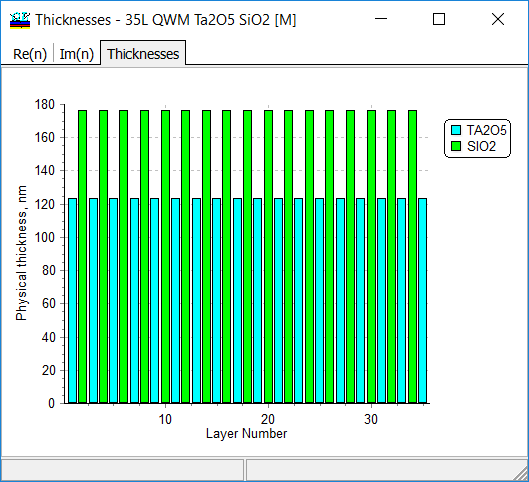Refractive index profile of the optimized design with reduced electric field intensity (EFI) inside the Ta2O5 layers: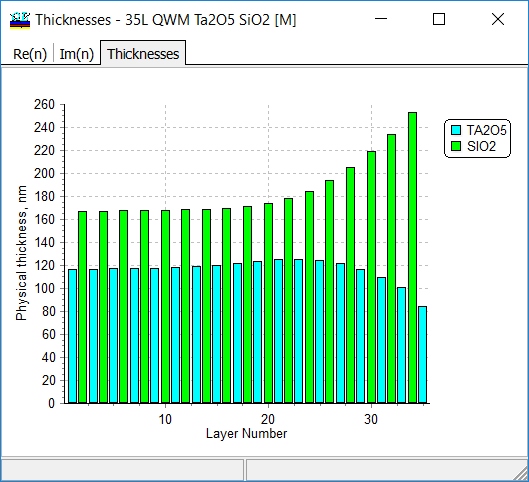Reflectance of the 35-layer Quarter-Wave Mirror: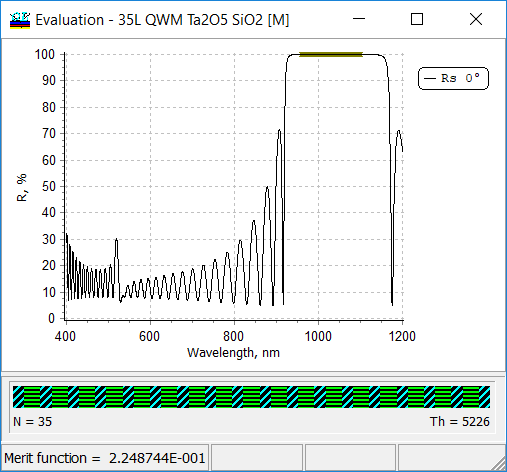Reflectance of the design with optimized electric-field intensity: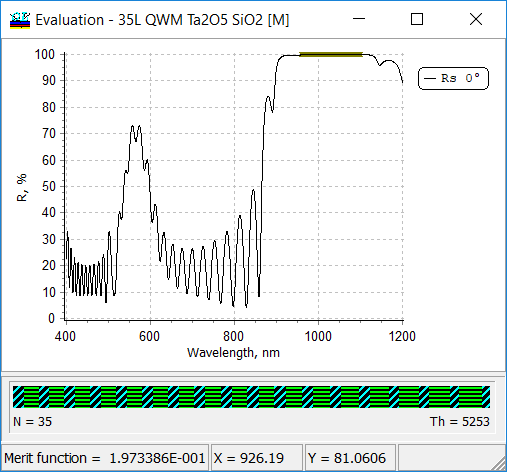Electric field distribution in the 35-layer quarter-wave stack: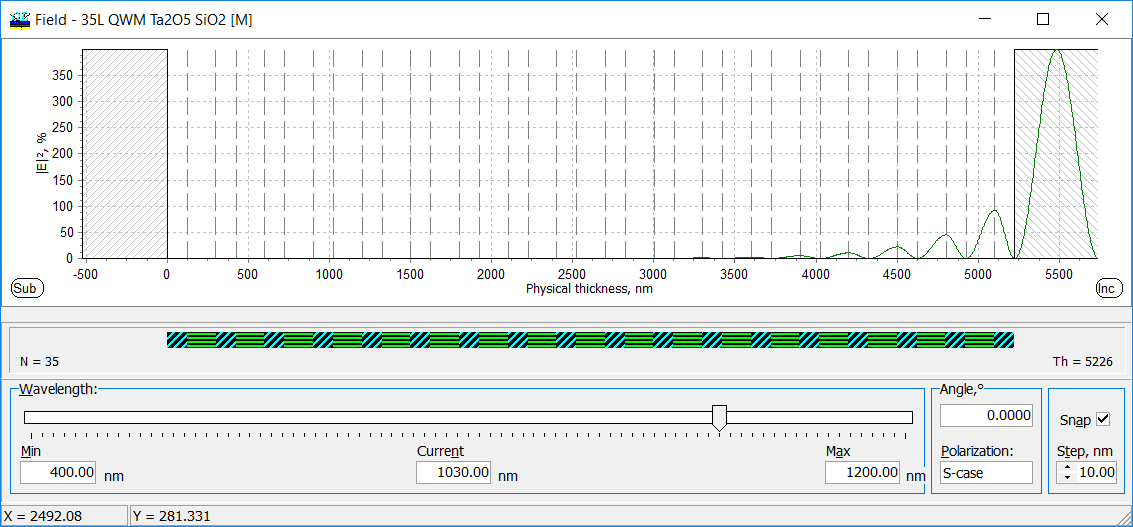Electric field in Ta2O5 layers is reduced: